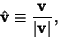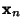## Unit Vector

A Vector of unit length. The unit vectorhaving the same direction as a given (nonzero) vector v is defined bywheredenotes the Norm of, is the unit vector in the same direction as the (finite) Vector v. A unit Vector in thedirection is given bywhereis the Radius Vector.